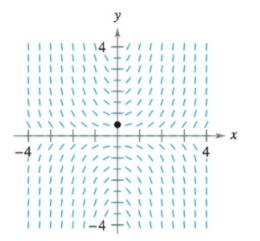Chapter 6.2, Problem 16E

Chapter
Section
Textbook Problem

Slope Field In Exercises 15 and 16, a differential equation, a point, and a slope field are given.(a) Sketch two approximate solutions of the differential equation on the slope field, one of which passes through the given point (To print an enlarged copy of the graph, go to MathGraphs.com.)(b) Use integration and the given point to find the particular solution of the differential equation and use a graphing utility to graph the solution. Compare the result with the sketch in part (a) that passes through the given point. d y d x = x y , ( 0 , 1 2 )(a)

To determine

To graph: For the differential equation dydx=xy the two approximate solutionand the point is (0,12).

Explanation

Given:

The differential equation is given as dydx=xy and the point is (0,12).

Graph:

Two approximate solutions of dydx=xy is:

 Value of dydx=xy Value of x Value of y dydx=0 0 0

(b)

To determine

To calculate: For the differential equation dydx=xy the solution at the given point is (0,12). Plot the graph of that solution using graphing utility. Provide comparison of the result with the that of part (a).

Still sussing out bartleby?

Check out a sample textbook solution.

See a sample solution

The Solution to Your Study Problems

Bartleby provides explanations to thousands of textbook problems written by our experts, many with advanced degrees!

Get Started

Find more solutions based on key concepts